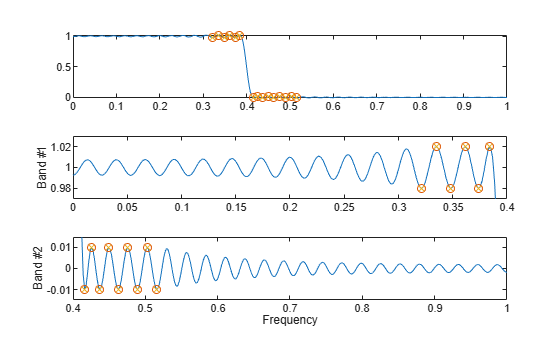# fircls

Constrained-least-squares FIR multiband filter design

## Syntax

```b = fircls(n,f,amp,up,lo) fircls(n,f,amp,up,lo,'design_flag') ```

## Description

`b = fircls(n,f,amp,up,lo)` generates a length `n+1` linear phase FIR filter `b`. The frequency-magnitude characteristics of this filter match those given by vectors `f` and `amp`:

• `f` is a vector of transition frequencies in the range from 0 to 1, where 1 corresponds to the Nyquist frequency. The first point of `f` must be `0` and the last point `1`. The frequency points must be in increasing order.

• `amp` is a vector describing the piecewise-constant desired amplitude of the frequency response. The length of `amp `is equal to the number of bands in the response and should be equal to `length(f)-1`.

• `up` and `lo` are vectors with the same length as `amp`. They define the upper and lower bounds for the frequency response in each band.

`fircls` always uses an even filter order for configurations with a passband at the Nyquist frequency (that is, highpass and bandstop filters). This is because for odd orders, the frequency response at the Nyquist frequency is necessarily 0. If you specify an odd-valued `n`, `fircls` increments it by 1.

`fircls(n,f,amp,up,lo,'design_flag')` enables you to monitor the filter design, where `'``design_flag``'` can be

• `'trace'`, for a textual display of the design error at each iteration step.

• `'plots'`, for a collection of plots showing the filter's full-band magnitude response and a zoomed view of the magnitude response in each sub-band. All plots are updated at each iteration step. The O's on the plot are the estimated extremals of the new iteration and the X's are the estimated extremals of the previous iteration, where the extremals are the peaks (maximum and minimum) of the filter ripples. Only ripples that have a corresponding O and X are made equal.

• `'both'`, for both the textual display and plots.

### Note

Normally, the lower value in the stopband will be specified as negative. By setting `lo` equal to `0` in the stopbands, a nonnegative frequency response amplitude can be obtained. Such filters can be spectrally factored to obtain minimum phase filters.

## Examples

collapse all

Design a 150th-order lowpass filter with a normalized cutoff frequency of $0.4\pi$ rad/sample. Specify a maximum absolute error of 0.02 in the passband and 0.01 in the stopband. Display plots of the bands.

```n = 150; f = [0 0.4 1]; a = [1 0]; up = [1.02 0.01]; lo = [0.98 -0.01]; b = fircls(n,f,a,up,lo,'both');```
``` Bound Violation = 0.0788344298966 ```
``` Bound Violation = 0.0096137744998 ```
``` Bound Violation = 0.0005681345753 ```
``` Bound Violation = 0.0000051519942 ```
``` Bound Violation = 0.0000000348656 `````` Bound Violation = 0.0000000006231 ```

The Bound Violations denote the iterations of the procedure as the design converges. Display the magnitude response of the filter.

`fvtool(b)`## Algorithms

`fircls` uses an iterative least-squares algorithm to obtain an equiripple response. The algorithm is a multiple exchange algorithm that uses Lagrange multipliers and Kuhn-Tucker conditions on each iteration.

## References

 Selesnick, I. W., M. Lang, and C. S. Burrus. “Constrained Least Square Design of FIR Filters without Specified Transition Bands.” Proceedings of the 1995 International Conference on Acoustics, Speech, and Signal Processing. Vol. 2, 1995, pp. 1260–1263.

 Selesnick, I. W., M. Lang, and C. S. Burrus. “Constrained Least Square Design of FIR Filters without Specified Transition Bands.” IEEE® Transactions on Signal Processing. Vol. 44, Number 8, 1996, pp. 1879–1892.